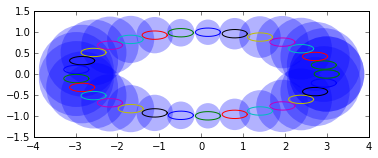# Condition number

In :
import numpy as np
import numpy.linalg as la
import matplotlib.pyplot as pt


Let's grab a $2\times 2$ matrix $A$:

In :
if 0:
np.random.seed(17)
A = np.random.randn(2, 2)
else:
A = np.array([[3, 0], [0,1]], dtype=np.float64)

A

Out:
array([[ 3.,  0.],
[ 0.,  1.]])


And its inverse:

In :
Ainv = la.inv(A)
Ainv

Out:
array([[ 0.33333333,  0.        ],
[ 0.        ,  1.        ]])


Now we would like to figure out where that matrix puts all the vectors with 2-norm 1.

To do so, let's make an array of vectors with vectors with norm 1:

In :
phi = np.linspace(0, 2*np.pi, 30)
xs = np.array([
np.cos(phi),
np.sin(phi)
])

pt.gca().set_aspect("equal")
pt.plot(xs, xs, "x")
pt.grid()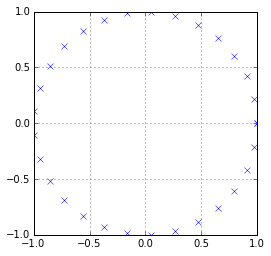Now apply $A$ to all those vectors...:

In :
Axs = A.dot(xs)
Axs.shape

Out:
(2, 30)


...and plot:

In :
pt.figure(figsize=(10, 5))

pt.subplot(121)
pt.title("$x$")
pt.plot(xs, xs, "x")
pt.gca().set_aspect("equal")

pt.subplot(122)
pt.title("$Ax$")
pt.plot(Axs, Axs, "v")
pt.gca().set_aspect("equal")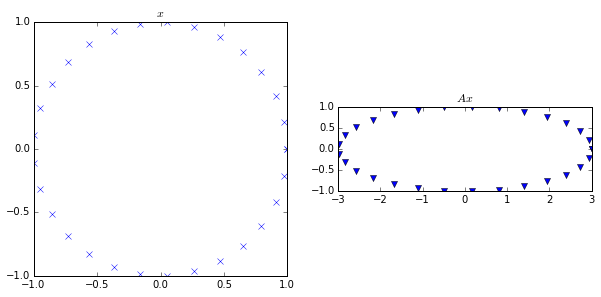Next, let's see what happens to small perturbations at each of the $x$ and $Ax$ points.

To that end, let's make an array ys of shape $2\times N_p\times N_p$, where $N_p$ is the number of points above.

In :
# ys has axes: XY x Npoints x Npoints

perturbation_size = 0.1
ys = perturbation_size * xs.reshape(2, -1, 1) + xs.reshape(2, 1, -1)

Ays = np.tensordot(A, ys, axes=1)
Ays.shape

Out:
(2, 30, 30)


Side note: What does the argument -1 to reshape do?

Let's plot what we've just made

In :
pt.figure(figsize=(10, 5))

pt.subplot(121)
pt.title("$y$")
pt.plot(ys, ys)
pt.gca().set_aspect("equal")

pt.subplot(122)
pt.title("$Ax$")
pt.plot(Ays, Ays)
pt.gca().set_aspect("equal")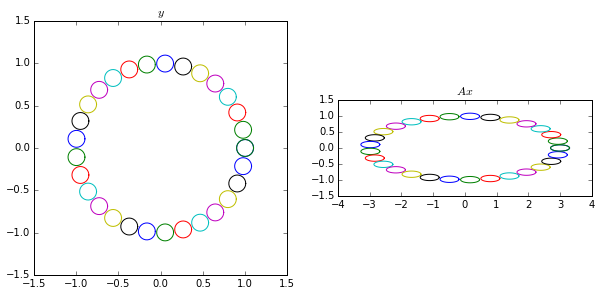Let's compare this with $\|A\|$:

In :
norm = la.norm(A, 2)
print(norm)

pt.plot(Ays, Ays)

ax = pt.gca()
ax.set_aspect("equal")

3.0


Out:
<matplotlib.patches.Circle at 0x7f35b11305c0>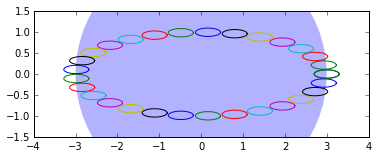What we want now is a circle around each of the $Ax$ that says,

"Because of the $\Delta x$ variation, $b$ is at most going to wiggle by this much, i.e. $\Delta b$ will be at most this big."

Now we want a $\kappa$ with $\frac{\|\Delta b\|}{\|b\|}\le \kappa \frac{\|\Delta x\|}{\|x\|}$.

Assume $\|x\|=1$. Equivalent: $\|\Delta b\|\le \kappa \|\Delta x\|\|b\|$.

Which $\kappa$ does the job?

In :
kappa = la.norm(A, 2)*la.norm(Ainv, 2)

In :
pt.plot(Ays, Ays)

ax = pt.gca()
ax.set_aspect("equal")
for i in range(Ays.shape):
b = Axs[:, i]
norm_delta_y = kappa * perturbation_size * la.norm(b)